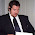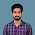## Friday, February 17, 2012

### Fibonacci Meets Pythagoras...

Here is an interesting pattern I found while substituting Fibonacci Numbers into the primitive solution for the Pythagorean problem.   The primitive solution to the Pythagorean problem is:

a2 + b2= c2
Given any two arbitrary integers  m  and   n
a =  n2  -  m2
b =  2mn
c =  m2  +  n2

I am not providing a full proof of this solution here. I am simply showing the solution because I use the solution to generate some very interesting patterns. I used Fibonacci numbers in the primitive solution and got the following results.... ↓ ↓ ↓ ↓ ↓ ↓ ↓Some of the Pythagorean triples from the chart are:
32  +  42 = 52
52  + 122 = 132    Look at the c terms do you see a pattern?.......                                                                  162 + 302 = 342     That's right the c terms are all Fibonacci numbers.                                                          392 + 802 = 892

If you look in the columns under the  m and the n you will notice that I have the Fibonacci sequence written in two ways the m column I started the Fibonacci sequence with 0 which still works with the pattern, and in the n column I have the Fibonacci sequence starting with 1.  I do this so when I substitute the numbers into the primitive Pythagorean solution They wont just zero out.

There is also another interesting pattern I found with the Fibonacci/Pythagorean triples.
If you look in the two columns on the right I have showed the place value the c terms are in the Fibonacci sequence. Example: the number 1 is the 2nd  number in the Fibonacci sequence and the number 2 is the 4th. And this is their place value when you start the Fibonacci sequence with 0.Using the column where I started the Fibonacci sequence  with 1 the corresponding c terms are in the odd place values instead.

To explain it another way here I will list out a few of the numbers from the c column

1. 1   is the 2nd Fibonacci number when the sequence starts with 0 and the 1st when it starts with 1
2. 2    is the 4th Fibonacci number when the sequence starts with 0 and the 3rd when it starts with 1
3. 5      is the 6th Fibonacci number when the sequence starts with 0 and the 5th when it starts with 1
4. 13    is the 8th Fibonacci number when the sequence starts with 0 and the 7th when it starts with 1
5. 34    is the 10th Fibonacci number when the sequence starts with 0 and the 9th when it starts with 1
6. 89  is the 12th Fibonacci number when the sequence starts with 0 and the 11th when it starts with 1
7. 233 is the 14th Fibonacci number when the sequence starts with 0 and the 15th when it starts with1

I could probably explain this better,but in general most should be able to look at the chart and see the patterns.  There is also more patterns found here when doing this.  The one I like is the c column has a lot of prime numbers. a few of them are     2,    5,   13,  89,    233,    1,597,   28,657.
I have carried this out as far as Excel will let me without throwing errors and all of the c terms are Fibonacci Numbers
I am curious to see what patterns the rest of you come up with.     Happy Hunting!!!!!!!!!!

1.In my opinion the explanation for pythagorean triple provided about by you is bit lengthy,So I am posting some simple definition and explanation about pythagorean triple that is Pythagorean triple is a set of three non-zero numbers in which the sum of the squares of two numbers is equal to the square of the third number. To explain this I am giving an example- set (5, 12, 13) is a Pythagorean triple,when
52 + 122 = 25 + 144 = 169
132 = 169

1.This post is an explanation of the patterns found while using Fibonacci numbers in the primitive solution to the Pythagorean problem. I put this together with an assumption of the basic understanding of what a Pythagorean triple was. I have actually not provided an explanation of Pythagorean triples at all.
However I do appreciate your input as to what Pythagorean triples are. Maybe your comment will help others reading this to have a little background on the subject.

2.i am so excited on your post,;i wish to hear your more explanation on it,,,can i have your any mail id plz???